## « Introduction to the Holonomic Gradient Method in Statistics

### Akimichi Takemura

The holonomic gradient method introduced by Nakayama et al. (2011) presents a new methodology for evaluating normalizing constants of probability distributions and for obtaining the maximum likelihood estimate of a statistical model. The method utilizes partial differential equations satisfied by the normalizing constant and is based on the Grobner basis theory for the ring of differential operators. In this talk we give an introduction to this new methodology. The method has already proved to be useful for problems in directional statistics and in classical multivariate distribution theory involving hypergeometric functions of matrix arguments.

Scroll with j/k | | | Size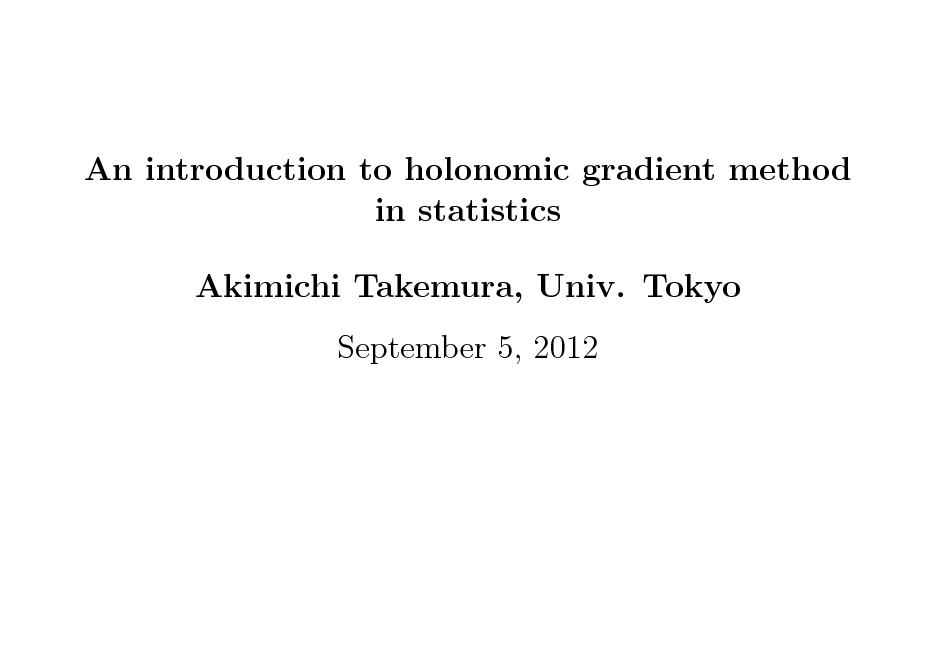An introduction to holonomic gradient method in statistics Akimichi Takemura, Univ. Tokyo September 5, 2012

1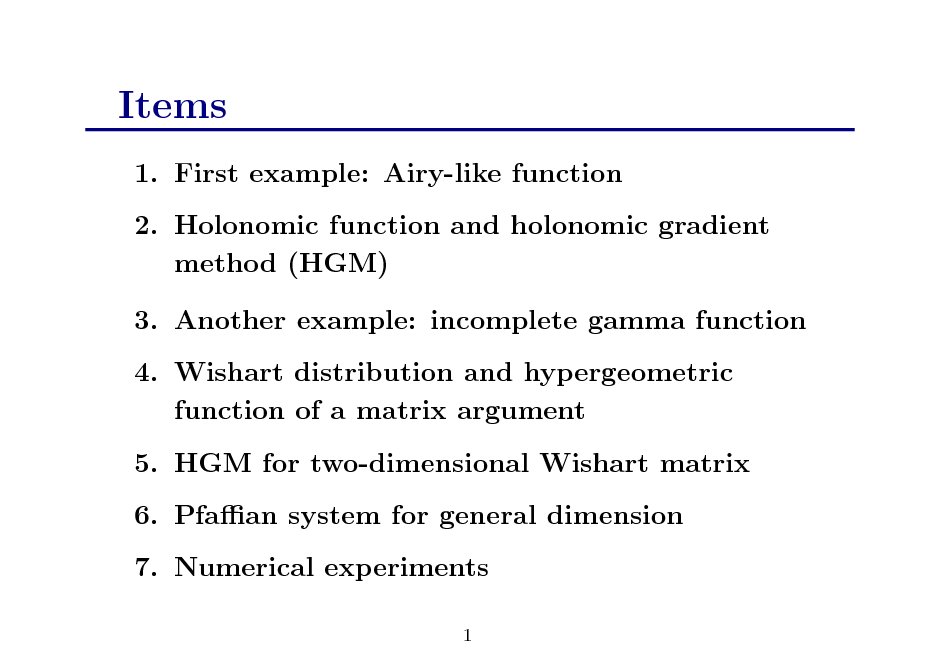Items
1. First example: Airy-like function 2. Holonomic function and holonomic gradient method (HGM) 3. Another example: incomplete gamma function 4. Wishart distribution and hypergeometric function of a matrix argument 5. HGM for two-dimensional Wishart matrix 6. Pfaan system for general dimension 7. Numerical experiments
1

2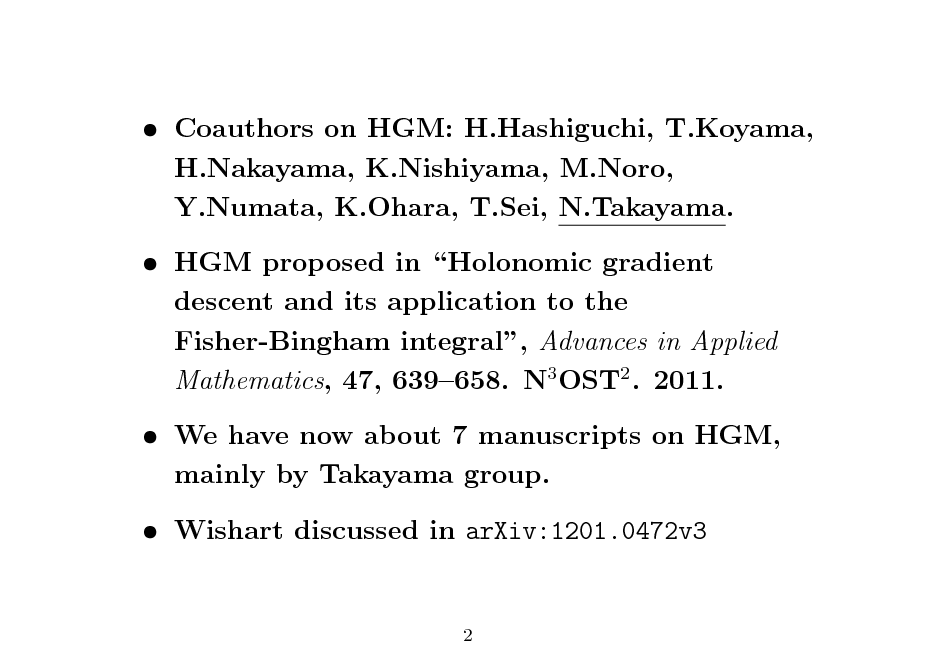Coauthors on HGM: H.Hashiguchi, T.Koyama, H.Nakayama, K.Nishiyama, M.Noro, Y.Numata, K.Ohara, T.Sei, N.Takayama. HGM proposed in Holonomic gradient descent and its application to the Fisher-Bingham integral, Advances in Applied Mathematics, 47, 639658. N3 OST2 . 2011. We have now about 7 manuscripts on HGM, mainly by Takayama group. Wishart discussed in arXiv:1201.0472v3
2

3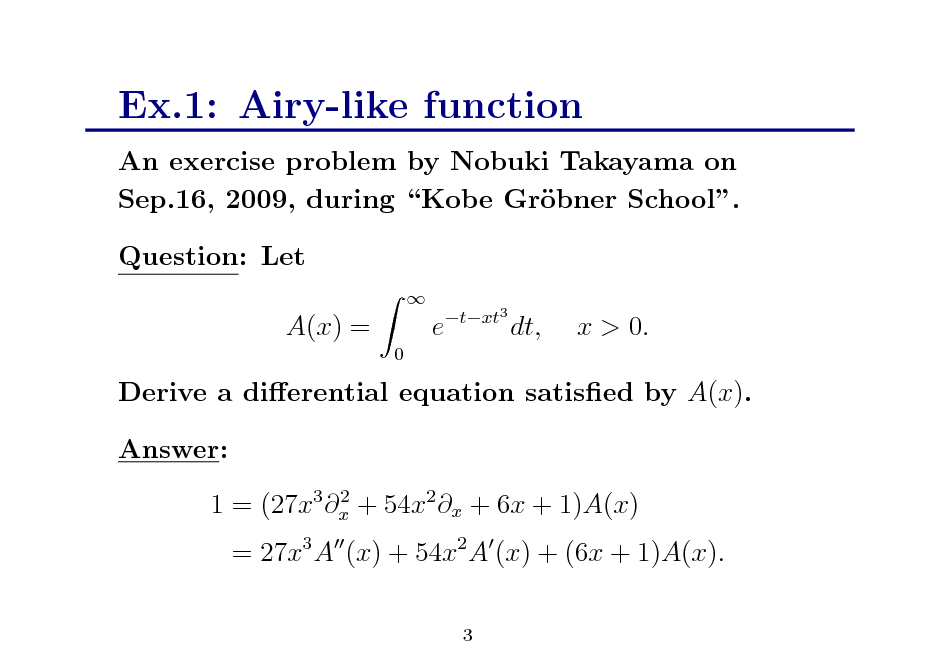Ex.1: Airy-like function
An exercise problem by Nobuki Takayama on Sep.16, 2009, during Kobe Grbner School. o Question: Let A(x) =
0

e

txt3

dt,

x > 0.

Derive a dierential equation satised by A(x). Answer:
2 1 = (27x3 x + 54x2 x + 6x + 1)A(x)

= 27x3 A (x) + 54x2 A (x) + (6x + 1)A(x).
3

4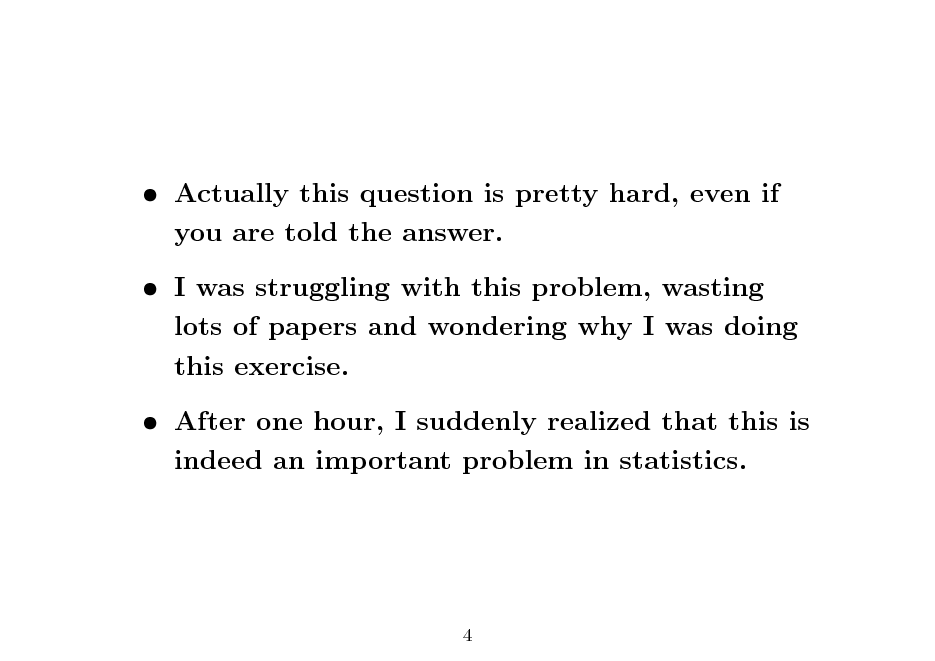Actually this question is pretty hard, even if you are told the answer. I was struggling with this problem, wasting lots of papers and wondering why I was doing this exercise. After one hour, I suddenly realized that this is indeed an important problem in statistics.

4

5We try to conrm the given answer. First A (x) = x A (x) =
0 0

e

txt3

dt =

0

te

3 txt3

dt

te

6 txt3

dt.

Hence 27x3 A (x) + 54x2 A (x) + (6x + 1)A(x) =
0 3 6 2 3 txt3

(27x t 54x t + 6x + 1)e

dt

= 1 ??

5

6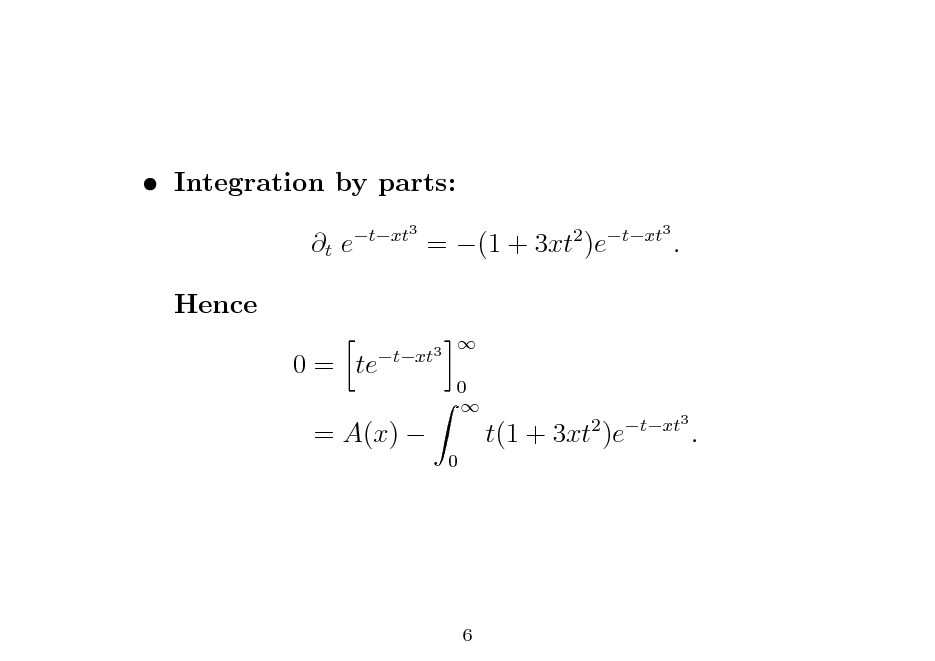Integration by parts: t e Hence 0 = te
txt3 0 0 txt3

= (1 + 3xt )e

2

txt3

.

= A(x)

t(1 + 3xt )e

2

txt3

.

6

7Similarly, if you work hard and work out 1 = (9x t + 3xt + 6xt 1)e
2 4 2 txt3 0

by integration by parts (how did you get this?), you obtain the answer:
0

(27x t 54x t + 6x + 1)e

3 6

2 3

txt3

dt = 1.

Believe and try (9x2 t4 + 3xt2 + 6xt 1) + (9x2 t4 3xt2 6xt + 1)(1 + 3xt2 ) = (36x2 t3 + 6xt + 6x) + (9x2 t4 3xt2 6xt + 1) + (27x3 t6 9x2 t4 18x2 t3 + 3xt2 ) (OK!)

= 27x3 t6 54x2 t3 + 6x + 1

7

8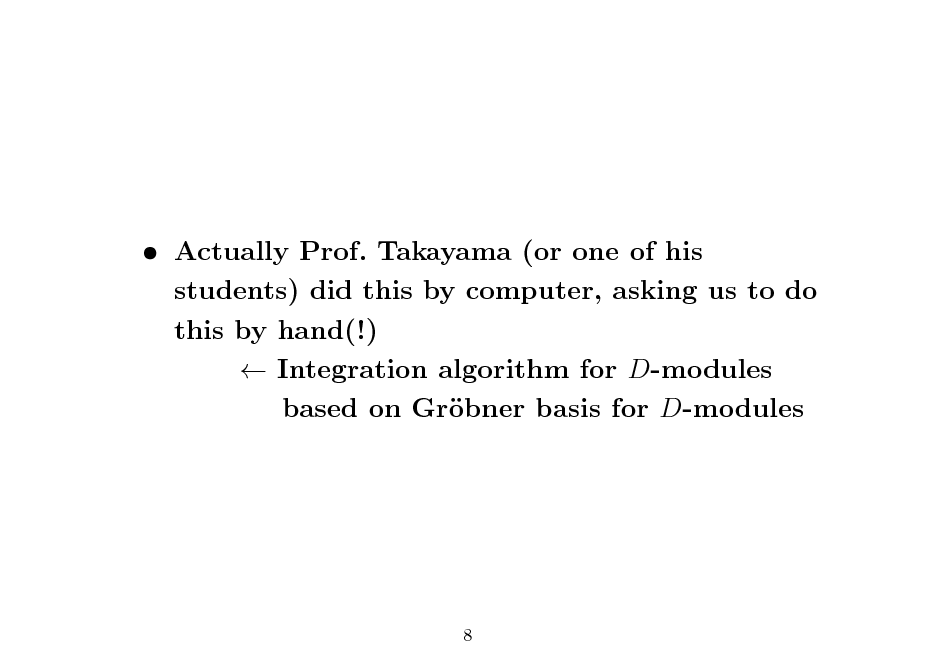Actually Prof. Takayama (or one of his students) did this by computer, asking us to do this by hand(!) Integration algorithm for D-modules based on Grbner basis for D-modules o

8

9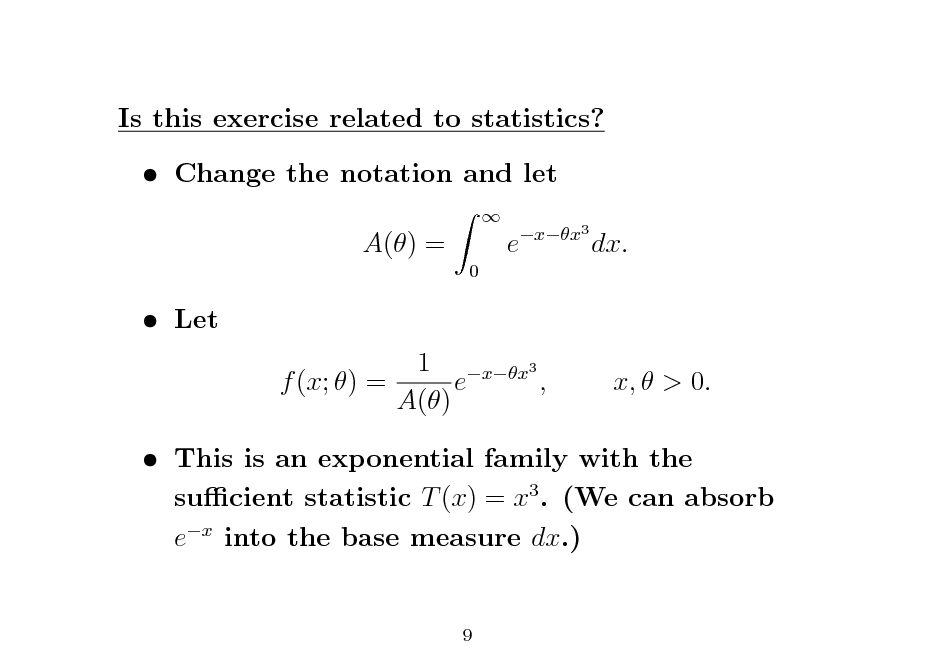Is this exercise related to statistics? Change the notation and let A() =
0

e

xx3

dx.

Let

1 xx3 f (x; ) = e , A()

x, > 0.

This is an exponential family with the sucient statistic T (x) = x3 . (We can absorb ex into the base measure dx.)

9

10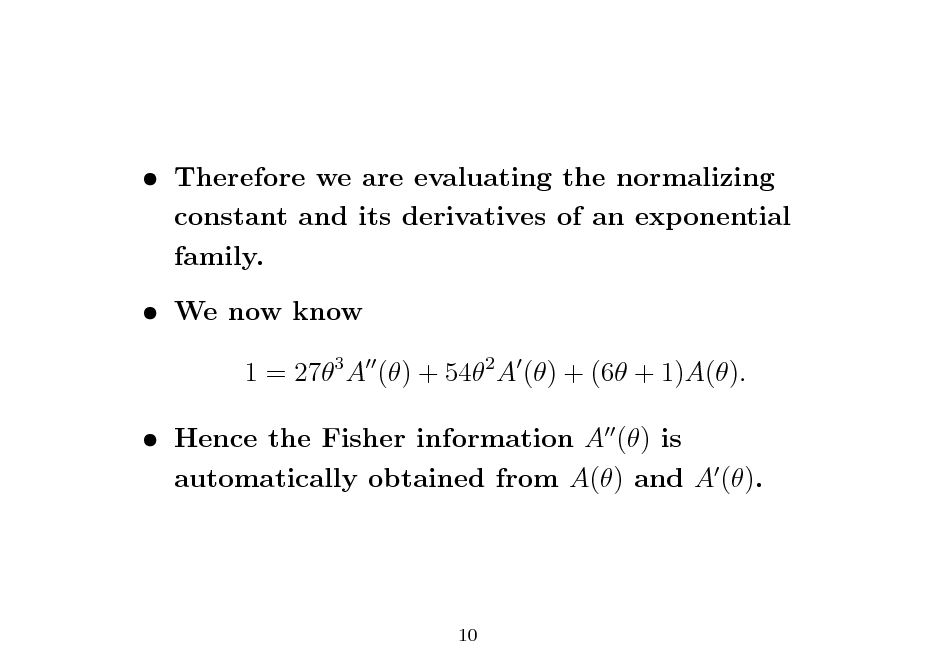Therefore we are evaluating the normalizing constant and its derivatives of an exponential family. We now know 1 = 273 A () + 542 A () + (6 + 1)A(). Hence the Fisher information A () is automatically obtained from A() and A ().

10

11Suppose that we have independent observations x1 , . . . , xn from f (x; ). Then the log likelihood () is written as
n

() =

i=1

x3 n log A() i

and the likelihood equation is
n

0=

x3 i
i=1

A () n . A()

Can we numerically evaluate A() and A ()? (Also A () for Newton-Raphson?)
11

12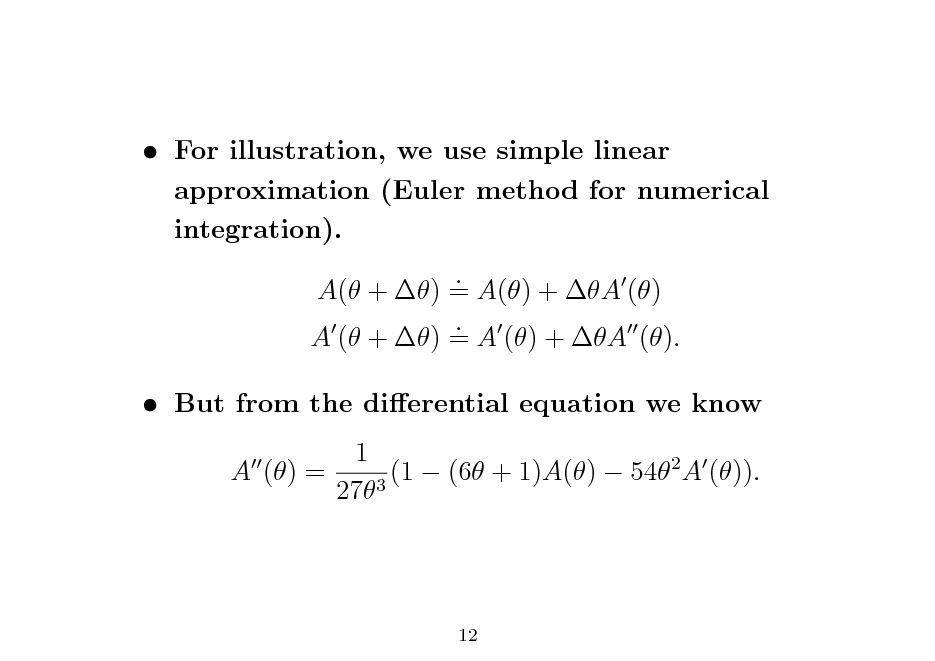For illustration, we use simple linear approximation (Euler method for numerical integration). . A( + ) = A() + A () . A ( + ) = A () + A (). But from the dierential equation we know 1 A () = (1 (6 + 1)A() 542 A ()). 273

12

13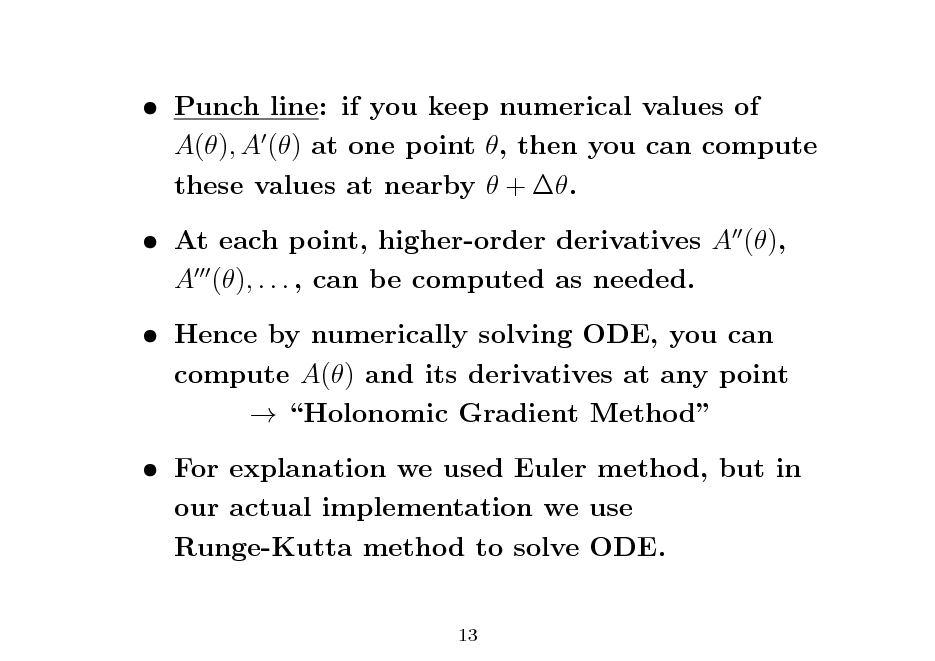Punch line: if you keep numerical values of A(), A () at one point , then you can compute these values at nearby + . At each point, higher-order derivatives A (), A (), . . . , can be computed as needed. Hence by numerically solving ODE, you can compute A() and its derivatives at any point Holonomic Gradient Method For explanation we used Euler method, but in our actual implementation we use Runge-Kutta method to solve ODE.
13

14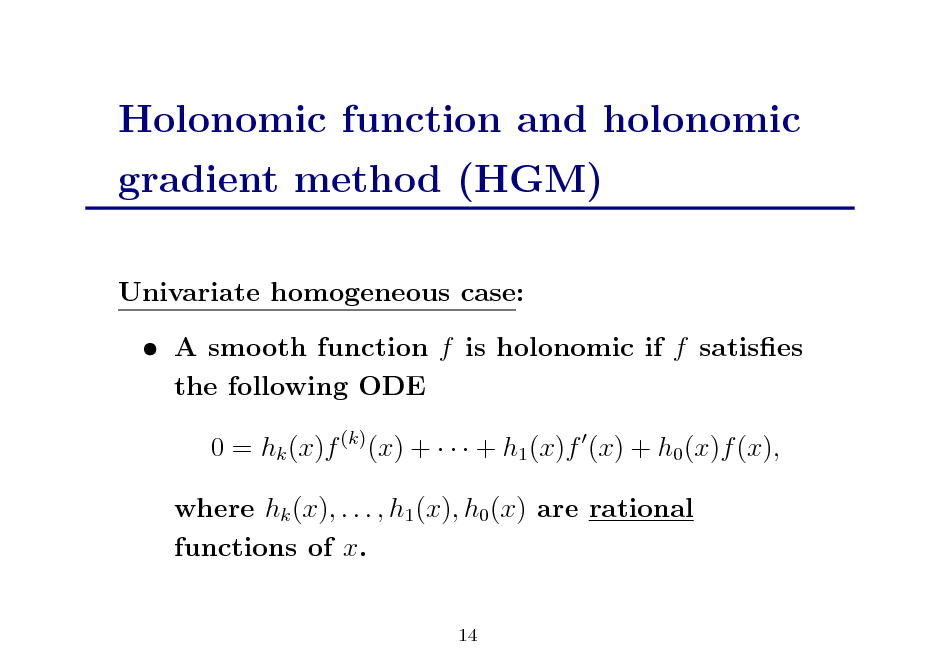Holonomic function and holonomic gradient method (HGM)
Univariate homogeneous case: A smooth function f is holonomic if f satises the following ODE 0 = hk (x)f (k) (x) + + h1 (x)f (x) + h0 (x)f (x), where hk (x), . . . , h1 (x), h0 (x) are rational functions of x.
14

15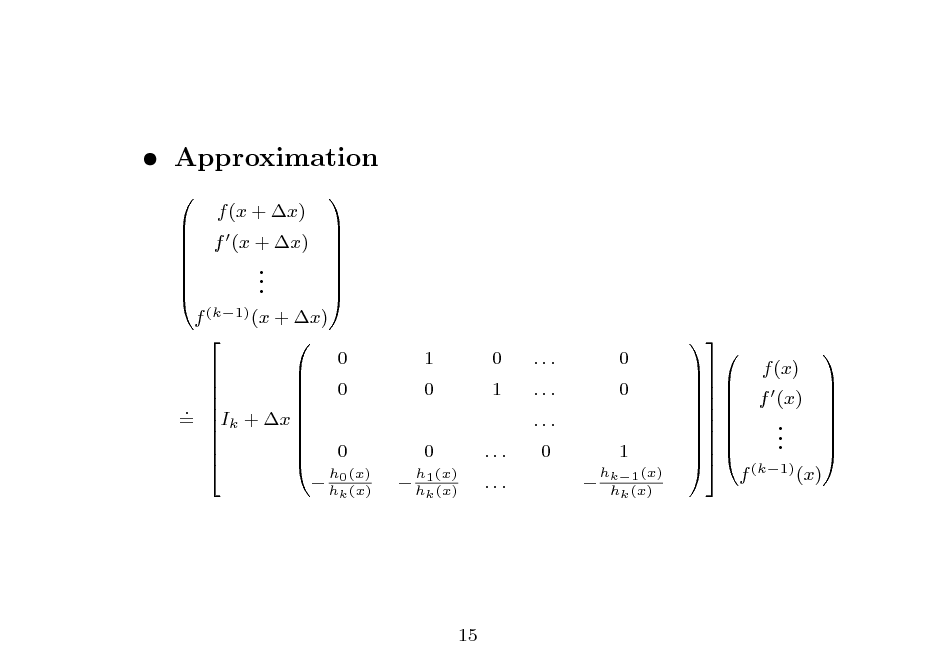Approximation
f (k1) (x + x) 0 0 . = Ik + x 0 f (x + x) . . .
k

f (x + x)

1 0 0 h1 (x)
k

0 1 ... ...

... ... ... 0

0 0 1
hk1 (x) hk (x)

h0 (x)

h (x)

h (x)

f (x) . . . f (k1) (x)

f (x)

15

16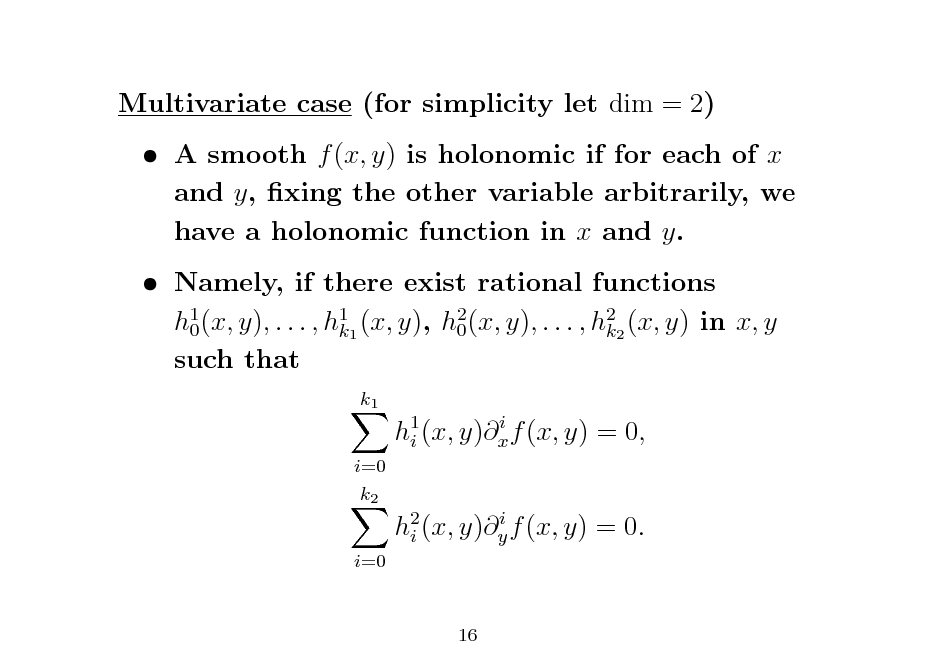Multivariate case (for simplicity let dim = 2) A smooth f (x, y) is holonomic if for each of x and y, xing the other variable arbitrarily, we have a holonomic function in x and y. Namely, if there exist rational functions h1 (x, y), . . . , h11 (x, y), h2 (x, y), . . . , h22 (x, y) in x, y 0 0 k k such that
k1 i h1 (x, y)x f (x, y) = 0, i i=0 k2 i h2 (x, y)y f (x, y) = 0. i i=0

16

17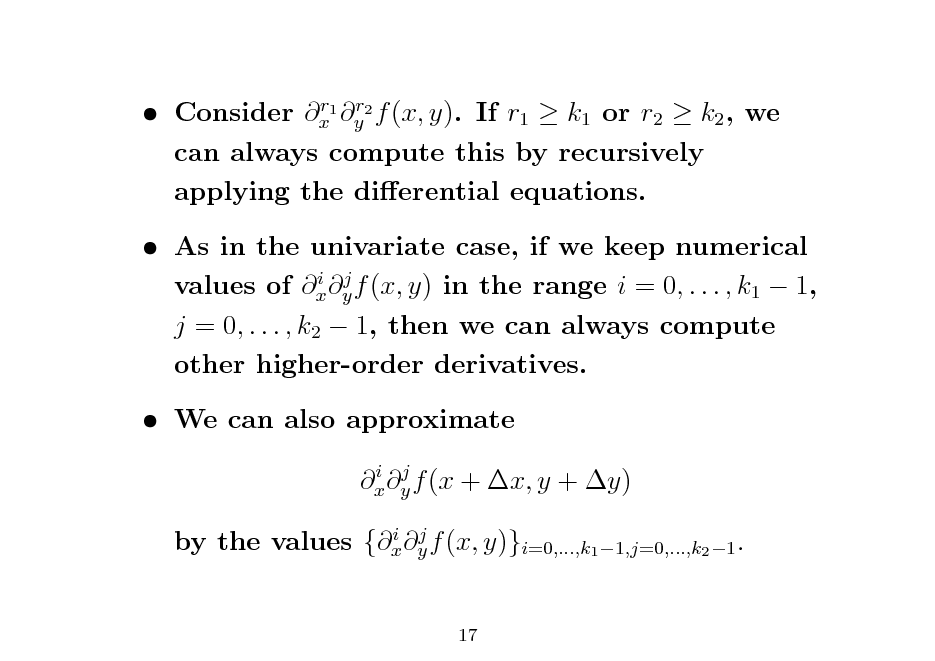r r Consider x1 y2 f (x, y). If r1 k1 or r2 k2 , we can always compute this by recursively applying the dierential equations.

As in the univariate case, if we keep numerical i j values of x y f (x, y) in the range i = 0, . . . , k1 1, j = 0, . . . , k2 1, then we can always compute other higher-order derivatives. We can also approximate
i j x y f (x + x, y + y) i j by the values {x y f (x, y)}i=0,...,k1 1,j=0,...,k2 1 .

17

18We usually only need to keep a subset of i j {x y f (x, y)}i=0,...,k1 1,j=0,...,k2 1 in memory. The subset is given by the set of standard monomials obtained by the division algorithm based on a Grbner basis. o

18

19Which functions are holonomic (Zeilberger(1990))? Polynomials and rational functions are holonomic. exp(rational), log(rational) are holonomic. f, g : holonomic f + g, f g : holonomic f (x1 , . . . , xm ) : holonomic f (x1 , . . . , xm )dxm : holonomic Restriction of a holonomic f to an ane subspace is holonomic.

19

20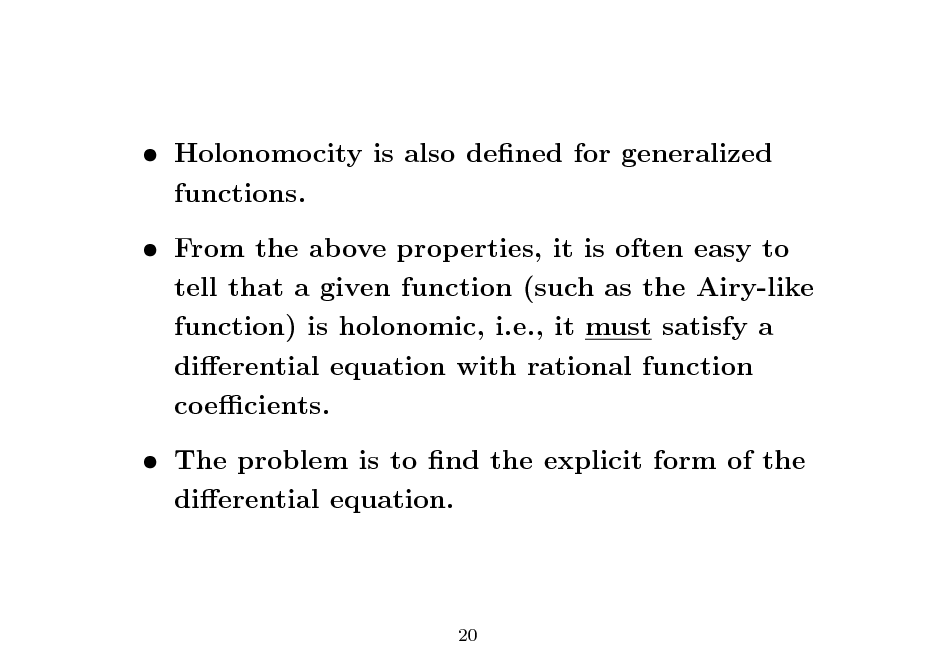Holonomocity is also dened for generalized functions. From the above properties, it is often easy to tell that a given function (such as the Airy-like function) is holonomic, i.e., it must satisfy a dierential equation with rational function coecients. The problem is to nd the explicit form of the dierential equation.

20

21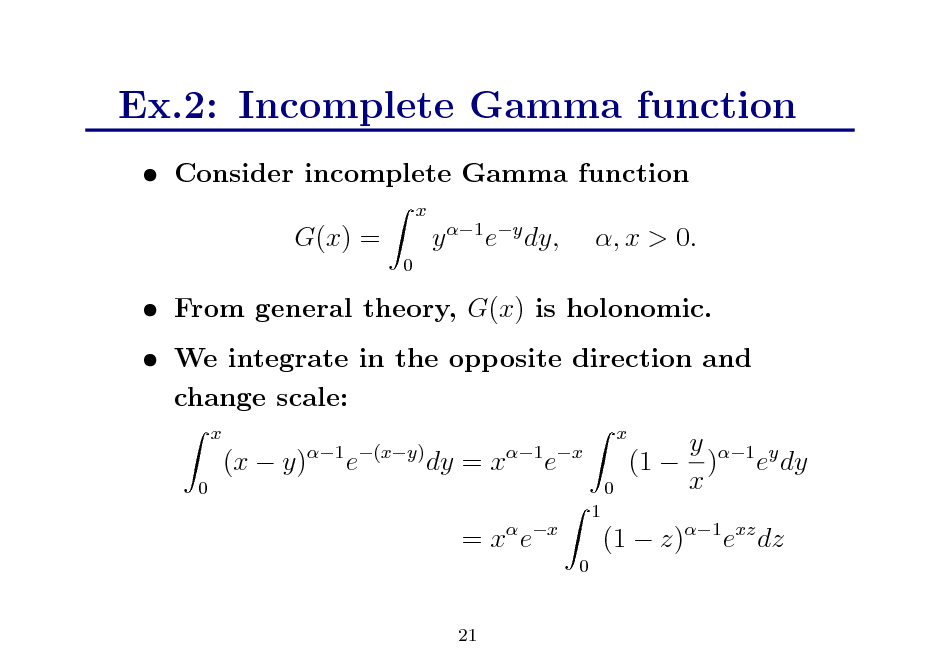Ex.2: Incomplete Gamma function
Consider incomplete Gamma function
x

G(x) =
0

y 1 ey dy,

, x > 0.

From general theory, G(x) is holonomic. We integrate in the opposite direction and change scale:
x 0

(x y)

1 (xy)

e

dy = x

1 x

x 0

e

y 1 y (1 ) e dy x

= x ex
0

1

(1 z)1 exz dz

21

22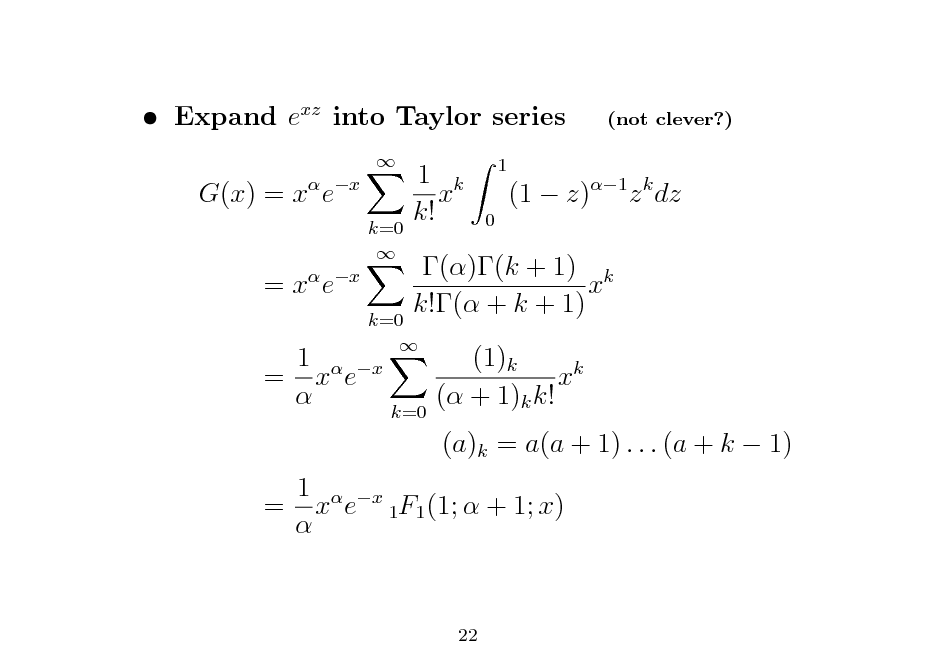Expand exz into Taylor series G(x) = x ex =x e
x k=0

(not clever?)

1 k x k!

1 0

(1 z)1 z k dz

1 = x e

k=0 x

()(k + 1) k x k!( + k + 1) (1)k xk ( + 1)k k! (a)k = a(a + 1) . . . (a + k 1)

k=0

1 x = x e 1F1 (1; + 1; x)

22

23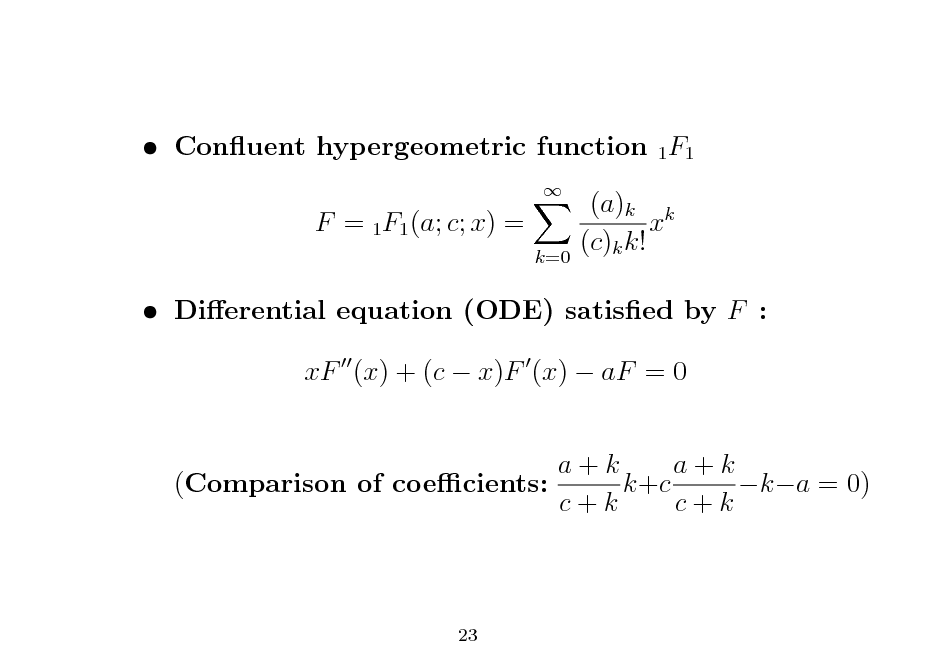Conuent hypergeometric function 1F1 F = 1F1 (a; c; x) =
k=0

(a)k k x (c)k k!

Dierential equation (ODE) satised by F : xF (x) + (c x)F (x) aF = 0 a+k a+k (Comparison of coecients: k+c ka = 0) c+k c+k

23

24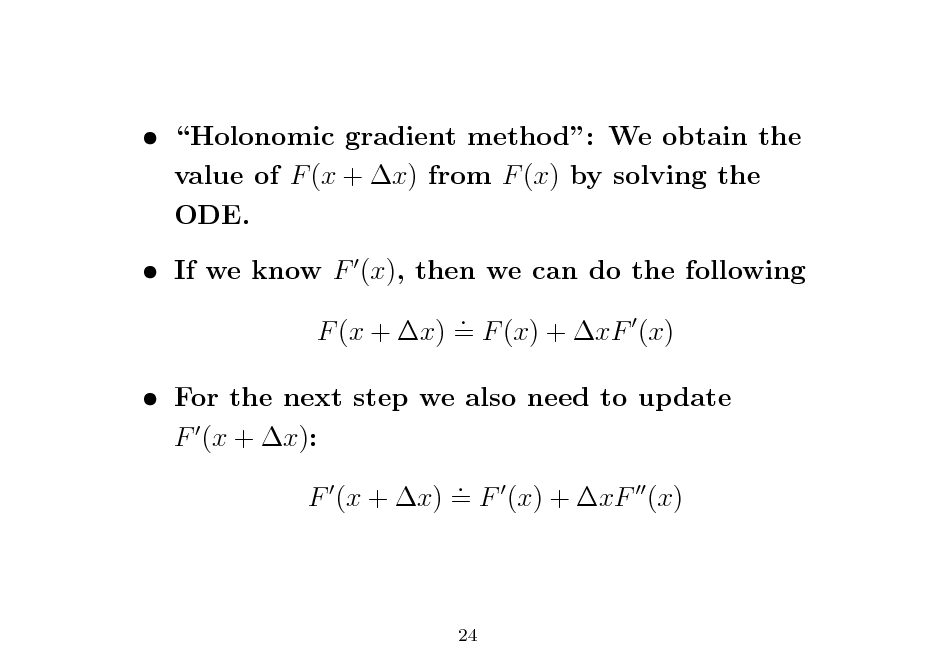Holonomic gradient method: We obtain the value of F (x + x) from F (x) by solving the ODE. If we know F (x), then we can do the following . F (x + x) = F (x) + xF (x)

For the next step we also need to update F (x + x): . F (x + x) = F (x) + xF (x)

24

25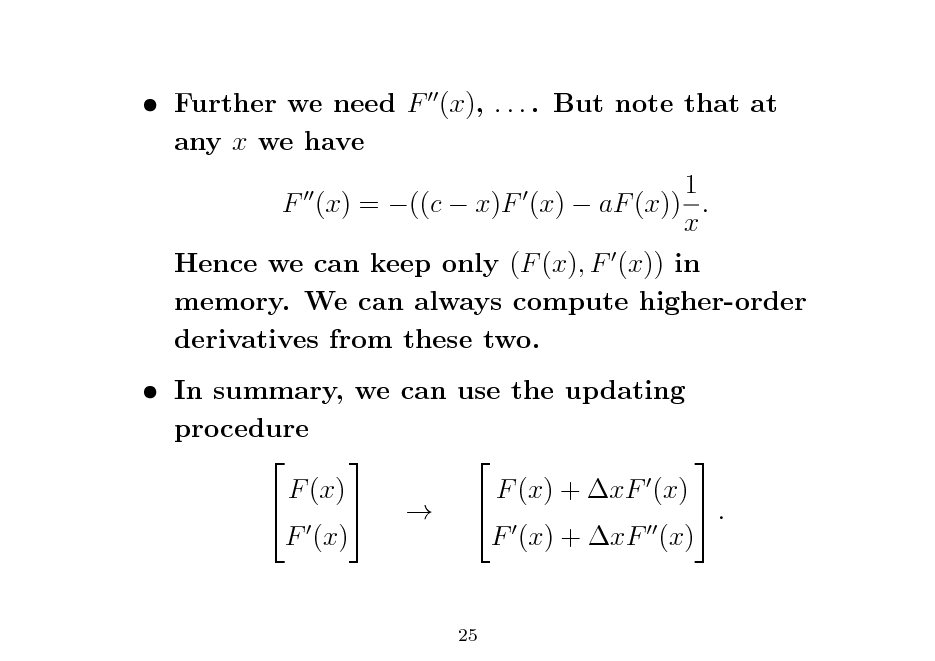Further we need F (x), . . . . But note that at any x we have 1 F (x) = ((c x)F (x) aF (x)) . x Hence we can keep only (F (x), F (x)) in memory. We can always compute higher-order derivatives from these two. In summary, we can use the updating procedure F (x) F (x) + xF (x) . F (x) F (x) + xF (x)
25

26In matrix form F (x) 0 1 F (x) + x . a F (x) cx F (x) x x How about the initial value? . expansion at x = 0.

Use the series

At x = 0 our ODE has singularity. Hence we have to step away from x = 0 by tiny amount. Generalization to matrix argument?

26

27Wishart distribution and hypergeometric function of a matrix argument
W : m m symmetric positive denite (W > 0) Density of Wishart distribution with d.f. n and covariance matrix > 0: |W | f (W ) = C n || 2
nm1 2

1 exp( trW 1 ) 2

C is known (containing gamma functions).
27

281

: the largest root of W
1

We want to evaluate the probability Pr(
1

< x).

< x W < xIm ,

where Im : m m is the identity matrix Hence the probability is given in the incomplete gamma form: Pr(
1

< x) = C
0<W <xIm

|W | n || 2
1

nm1 2

1 exp( trW 1 )dW 2

From general theory Pr(
28

< x) is holonomic.

29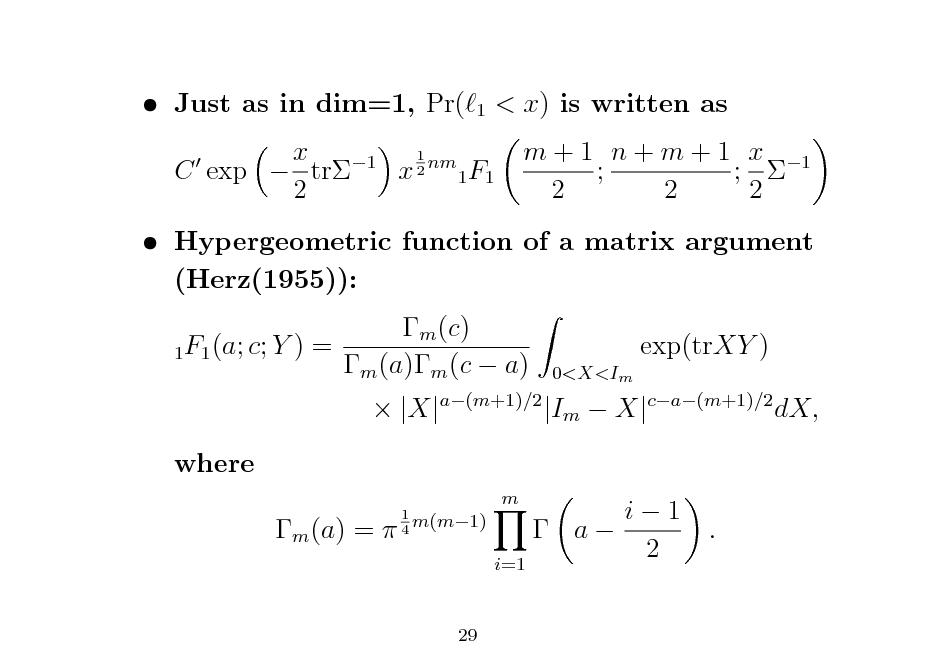Just as in dim=1, Pr(

1

< x) is written as m + 1 n + m + 1 x 1 ; ; 2 2 2

1 x 1 C exp tr x 2 nm 1F1 2

Hypergeometric function of a matrix argument (Herz(1955)): m (c) 1F1 (a; c; Y ) = m (a)m (c a) where m (a) =
1 m(m1) 4

exp(trXY )
0<X<Im

|X|a(m+1)/2 |Im X|ca(m+1)/2 dX, i1 a 2 i=1
m

.

29

30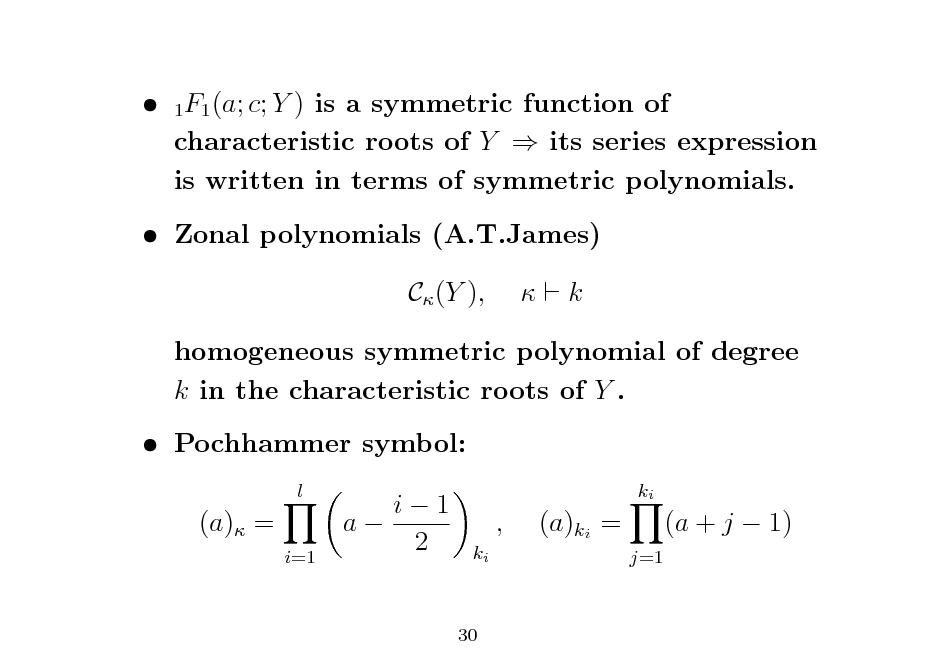1F1 (a; c; Y ) is a symmetric function of characteristic roots of Y its series expression is written in terms of symmetric polynomials. Zonal polynomials (A.T.James) C (Y ), k

homogeneous symmetric polynomial of degree k in the characteristic roots of Y . Pochhammer symbol:
l

(a) =
i=1

i1 a 2

ki

,
ki

(a)ki =
j=1

(a + j 1)

30

31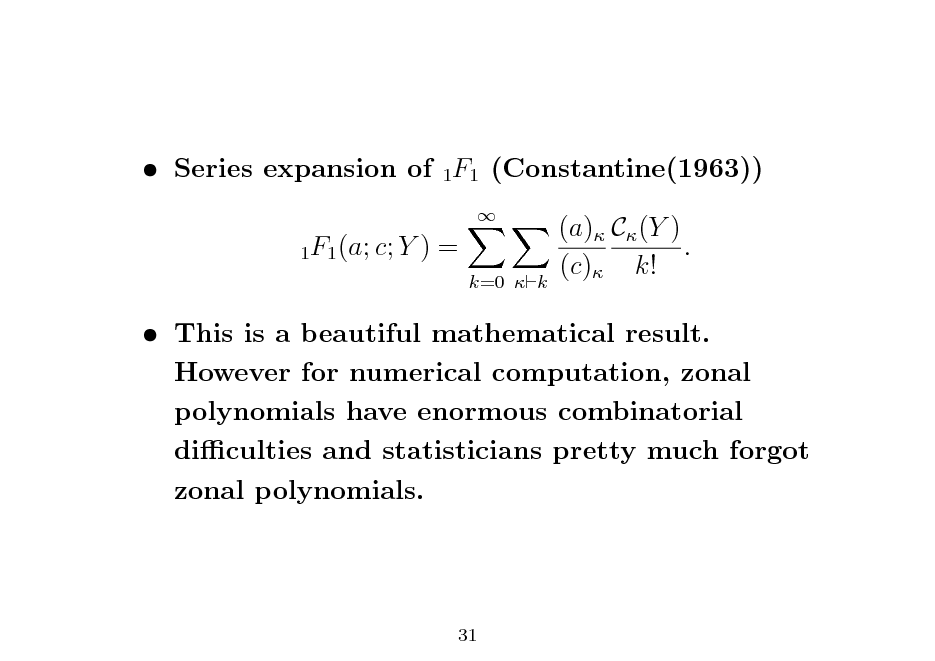Series expansion of 1F1 (Constantine(1963))
1F1 (a; c; Y

)=

k=0 k

(a) C (Y ) . (c) k!

This is a beautiful mathematical result. However for numerical computation, zonal polynomials have enormous combinatorial diculties and statisticians pretty much forgot zonal polynomials.

31

32The partial dierential equation satised by F (Y ) = 1F1 (a; c; y1 , . . . , ym ) was obtained by Muirhead(1970). gi F = 0, i = 1, . . . , m, where gi = yi i2 1 + (c yi )i + 2 yj (i j ) a. yi yj

j=i

32

33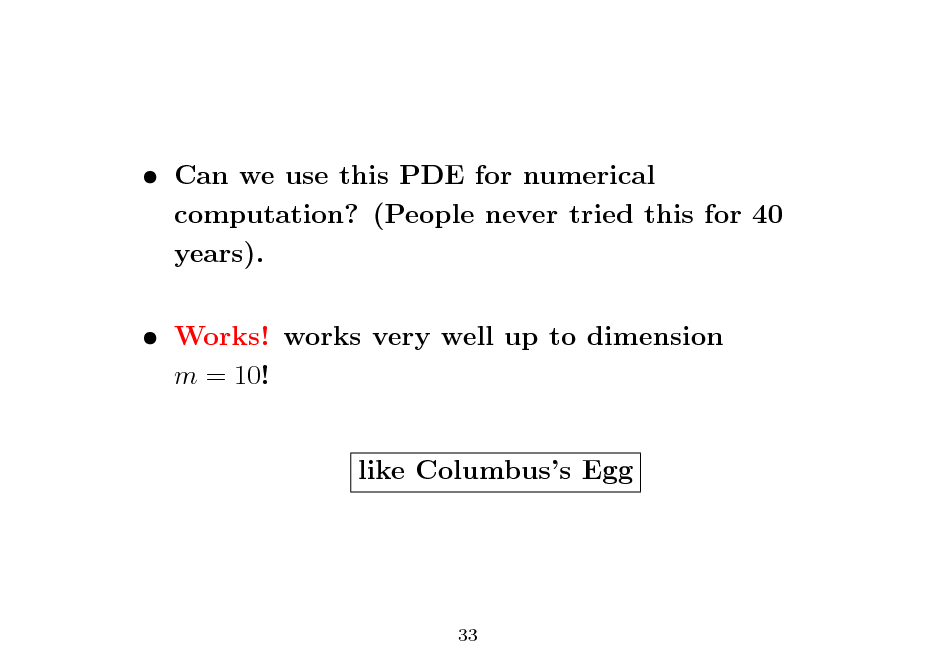Can we use this PDE for numerical computation? (People never tried this for 40 years). Works! works very well up to dimension m = 10! like Columbuss Egg

33

34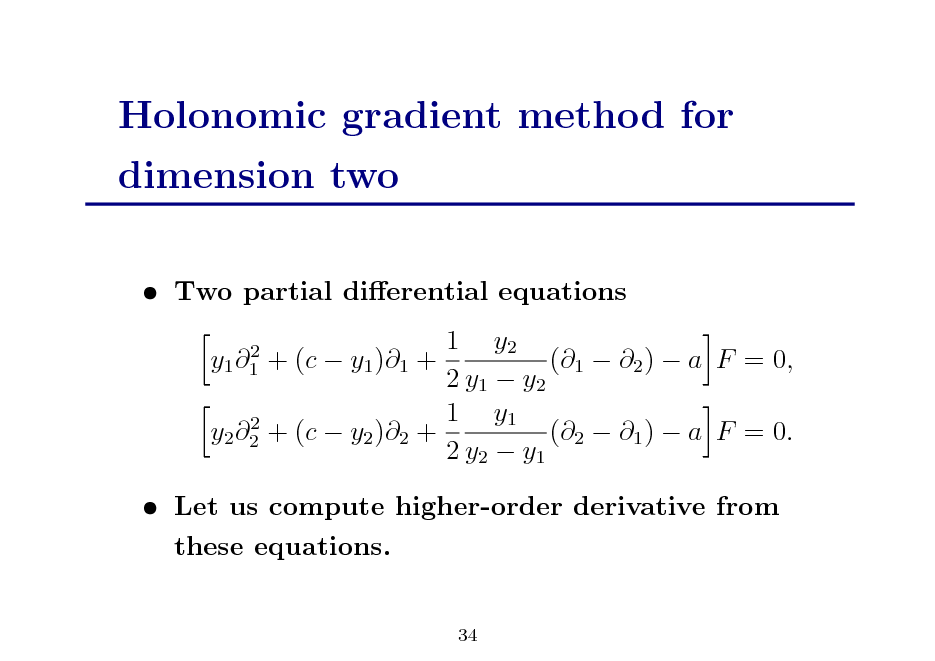Holonomic gradient method for dimension two
Two partial dierential equations 1 y2 + (c y1 )1 + (1 2 ) a F = 0, 2 y1 y2 1 y1 2 y2 2 + (c y2 )2 + (2 1 ) a F = 0. 2 y2 y1
2 y1 1

Let us compute higher-order derivative from these equations.
34

35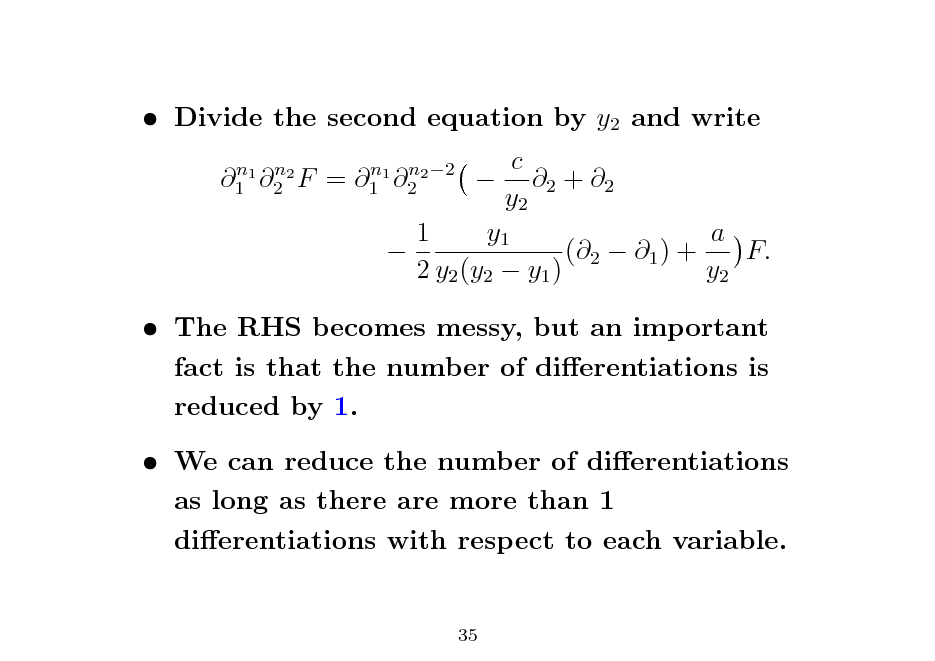Divide the second equation by y2 and write
n n 1 1 2 2 F

=

n n 1 1 2 2 2

c 2 + 2 y2 1 y1 a (2 1 ) + F. 2 y2 (y2 y1 ) y2

The RHS becomes messy, but an important fact is that the number of dierentiations is reduced by 1. We can reduce the number of dierentiations as long as there are more than 1 dierentiations with respect to each variable.

35

36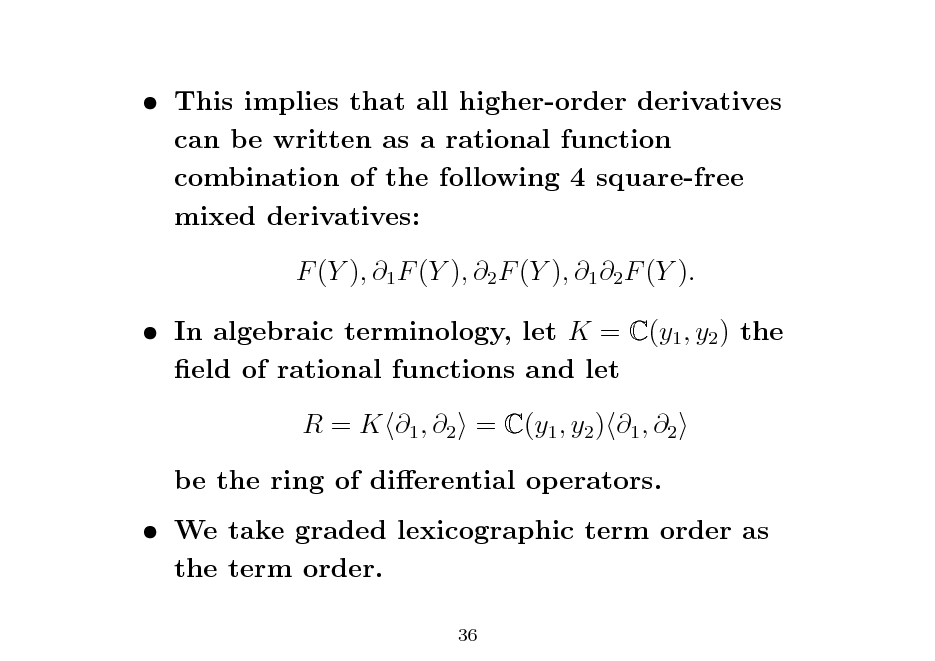This implies that all higher-order derivatives can be written as a rational function combination of the following 4 square-free mixed derivatives: F (Y ), 1 F (Y ), 2 F (Y ), 1 2 F (Y ). In algebraic terminology, let K = C(y1 , y2 ) the eld of rational functions and let R = K 1 , 2 = C(y1 , y2 ) 1 , 2 be the ring of dierential operators. We take graded lexicographic term order as the term order.
36

37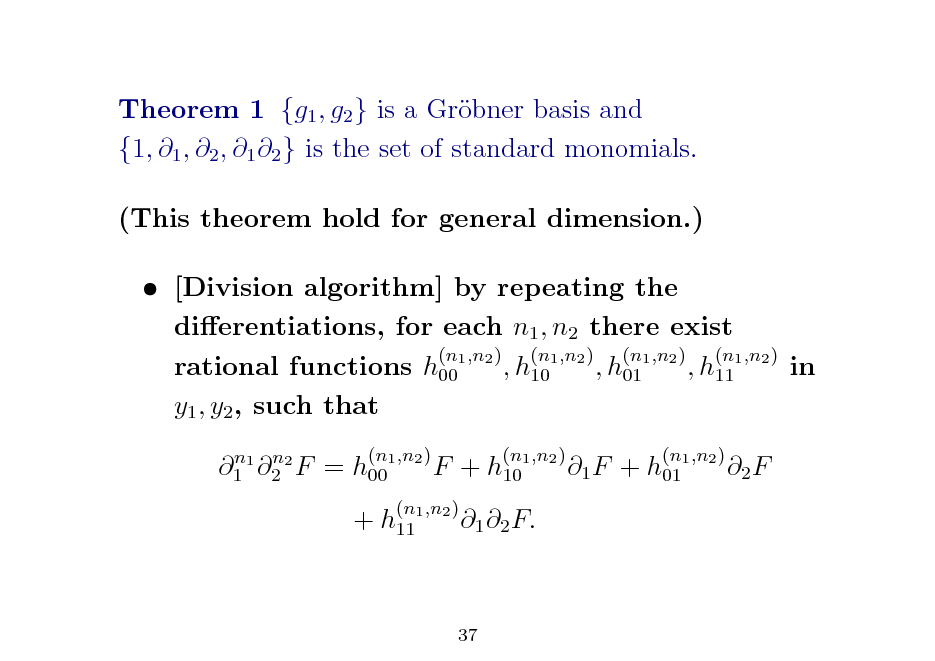Theorem 1 {g1 , g2 } is a Grbner basis and o {1, 1 , 2 , 1 2 } is the set of standard monomials. (This theorem hold for general dimension.) [Division algorithm] by repeating the dierentiations, for each n1 , n2 there exist (n ,n ) (n ,n ) (n ,n ) (n ,n ) rational functions h001 2 , h101 2 , h011 2 , h111 2 in y1 , y2 , such that
n n 1 1 2 2 F = h001 (n ,n2 )

F + h101

(n ,n2 )

1 F + h011

(n ,n2 )

2 F

+ h111

(n ,n2 )

1 2 F.

37

38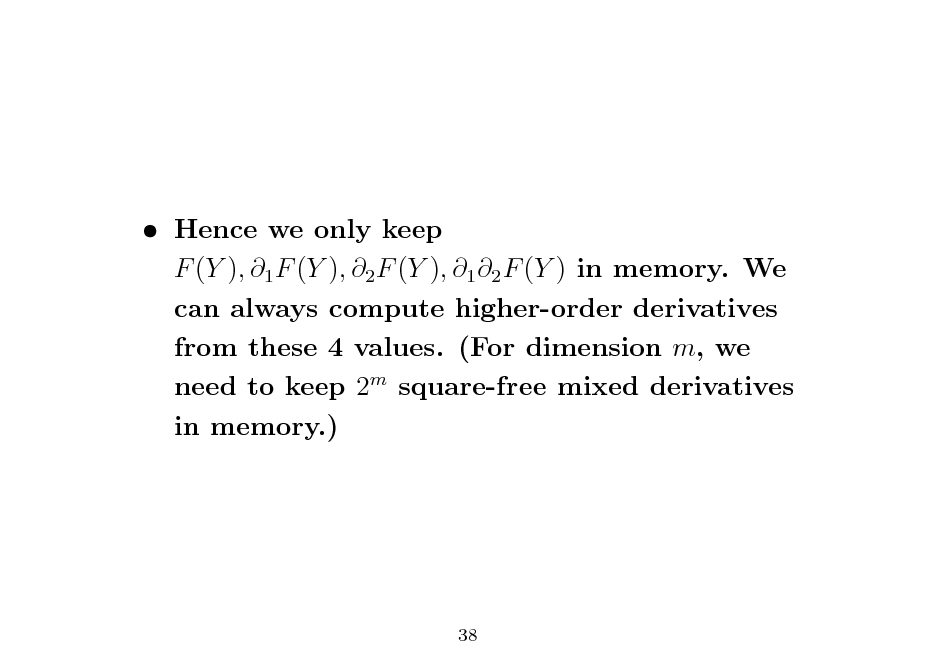Hence we only keep F (Y ), 1 F (Y ), 2 F (Y ), 1 2 F (Y ) in memory. We can always compute higher-order derivatives from these 4 values. (For dimension m, we need to keep 2m square-free mixed derivatives in memory.)

38

39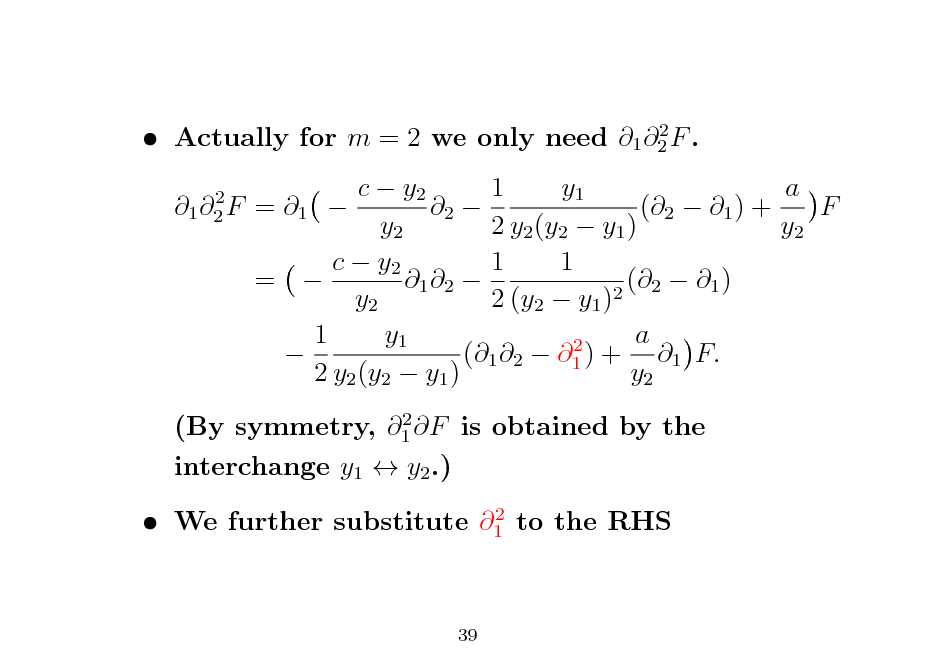2 Actually for m = 2 we only need 1 2 F . 2 1 2 F

c y2 1 y1 a = 1 2 (2 1 ) + F y2 2 y2 (y2 y1 ) y2 c y2 1 1 = 1 2 (2 1 ) 2 y2 2 (y2 y1 ) 1 y1 a 2 (1 2 1 ) + 1 F. 2 y2 (y2 y1 ) y2

2 (By symmetry, 1 F is obtained by the interchange y1 y2 .) 2 We further substitute 1 to the RHS

39

40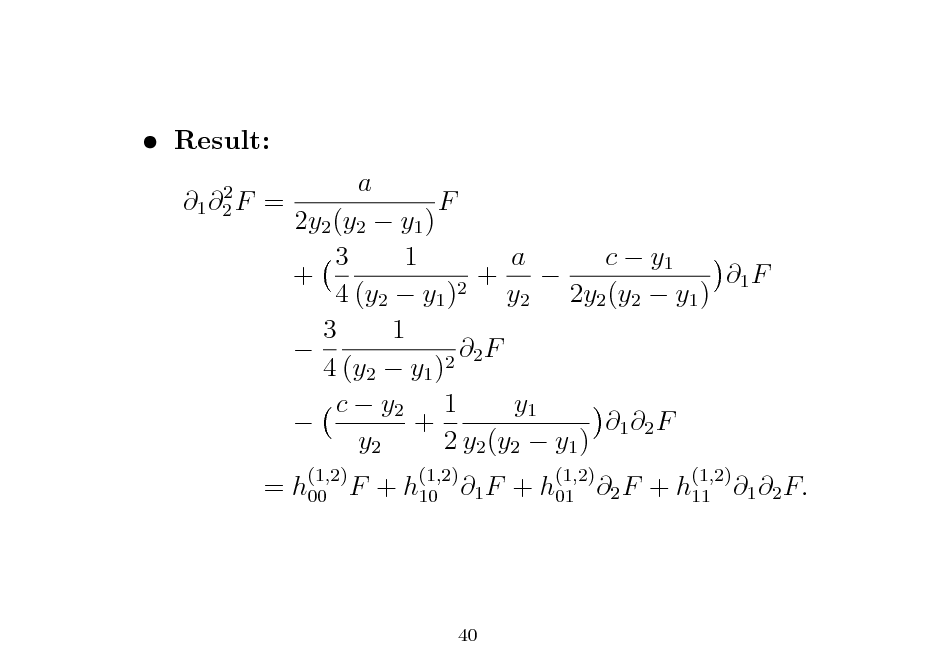Result:
2 1 2 F

=

a

= h00 F + h10 1 F + h01 2 F + h11 1 2 F.

2y2 (y2 y1 ) 3 1 a c y1 + + 1 F 2 4 (y2 y1 ) y2 2y2 (y2 y1 ) 3 1 F 2 2 4 (y2 y1 ) c y2 1 y1 + 1 2 F y2 2 y2 (y2 y1 )
(1,2) (1,2) (1,2) (1,2)

F

40

41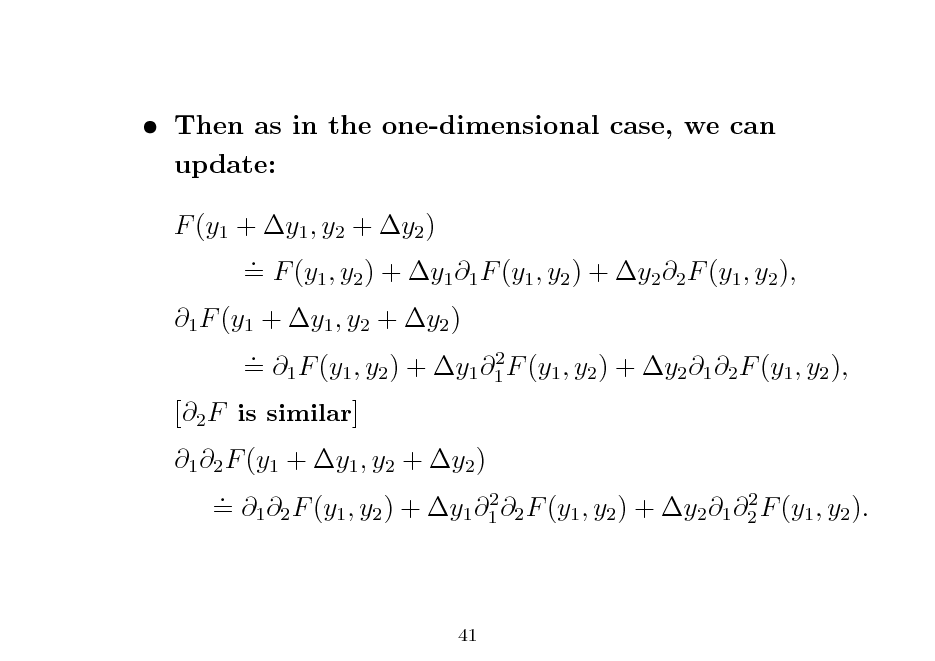Then as in the one-dimensional case, we can update: F (y1 + y1 , y2 + y2 ) . = F (y1 , y2 ) + y1 1 F (y1 , y2 ) + y2 2 F (y1 , y2 ), 1 F (y1 + y1 , y2 + y2 ) . 2 = 1 F (y1 , y2 ) + y1 1 F (y1 , y2 ) + y2 1 2 F (y1 , y2 ), [2 F is similar] 1 2 F (y1 + y1 , y2 + y2 ) . 2 2 = 1 2 F (y1 , y2 ) + y1 1 2 F (y1 , y2 ) + y2 1 2 F (y1 , y2 ).

41

42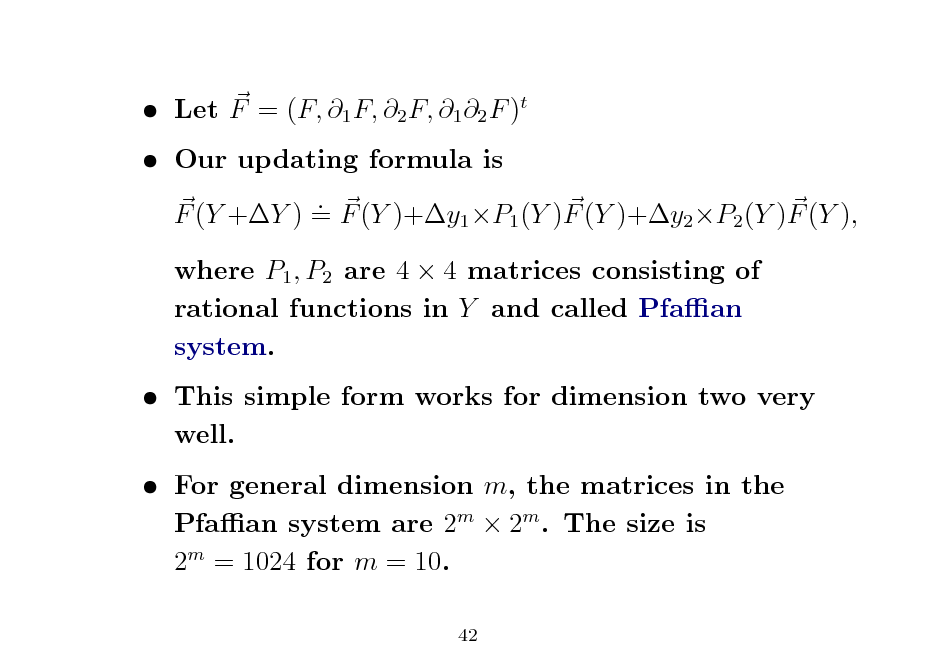Let F = (F, 1 F, 2 F, 1 2 F )t Our updating formula is . F (Y +Y ) = F (Y )+y1 P1 (Y )F (Y )+y2 P2 (Y )F (Y ), where P1 , P2 are 4 4 matrices consisting of rational functions in Y and called Pfaan system. This simple form works for dimension two very well. For general dimension m, the matrices in the Pfaan system are 2m 2m . The size is 2m = 1024 for m = 10.
42

43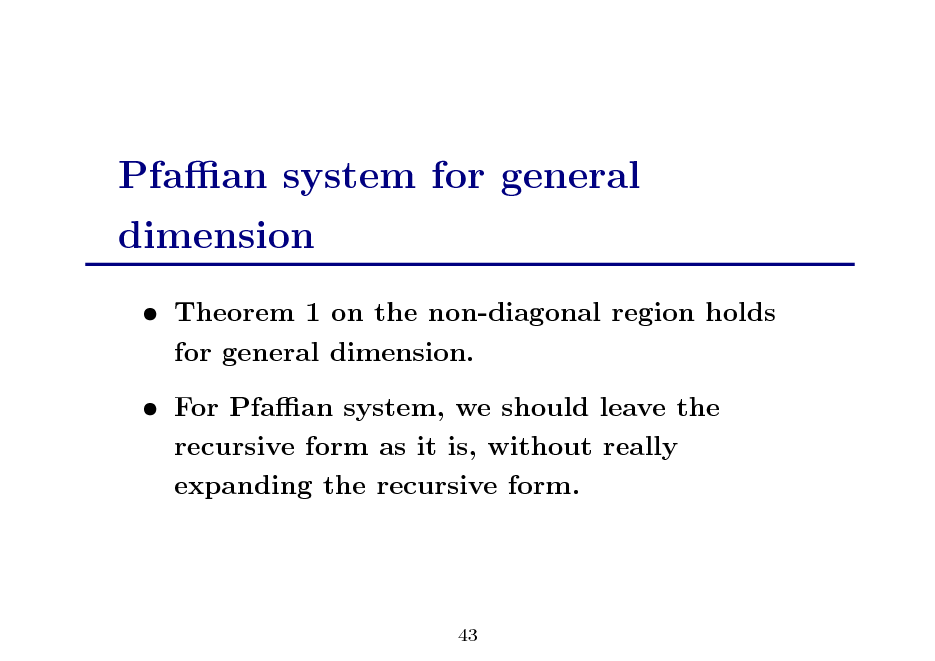Pfaan system for general dimension
Theorem 1 on the non-diagonal region holds for general dimension. For Pfaan system, we should leave the recursive form as it is, without really expanding the recursive form.

43

44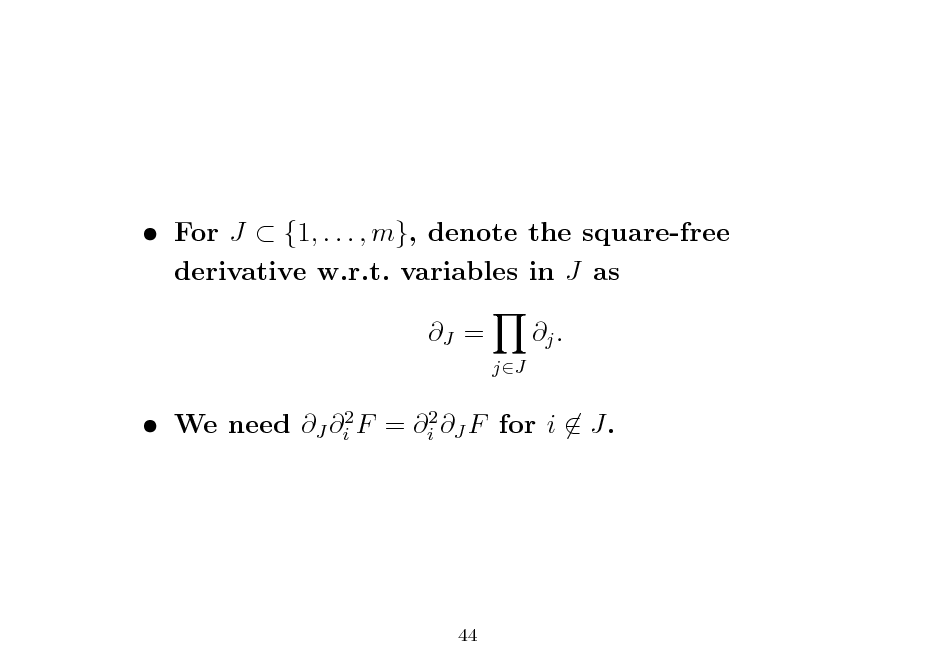For J {1, . . . , m}, denote the square-free derivative w.r.t. variables in J as J =
jJ

j .

We need J i2 F = i2 J F for i J.

44

45Let I = J {i}. Collect square-free terms in J gi as 1 r(i, J; y) = (c yi )I aJ + 2 1 + 2 1 + 2 yk I yi yk yk (I J k ) yi yk

kI

kJ

kJ

yi (i J\{k} J ) . 2 (yi yk )

45

46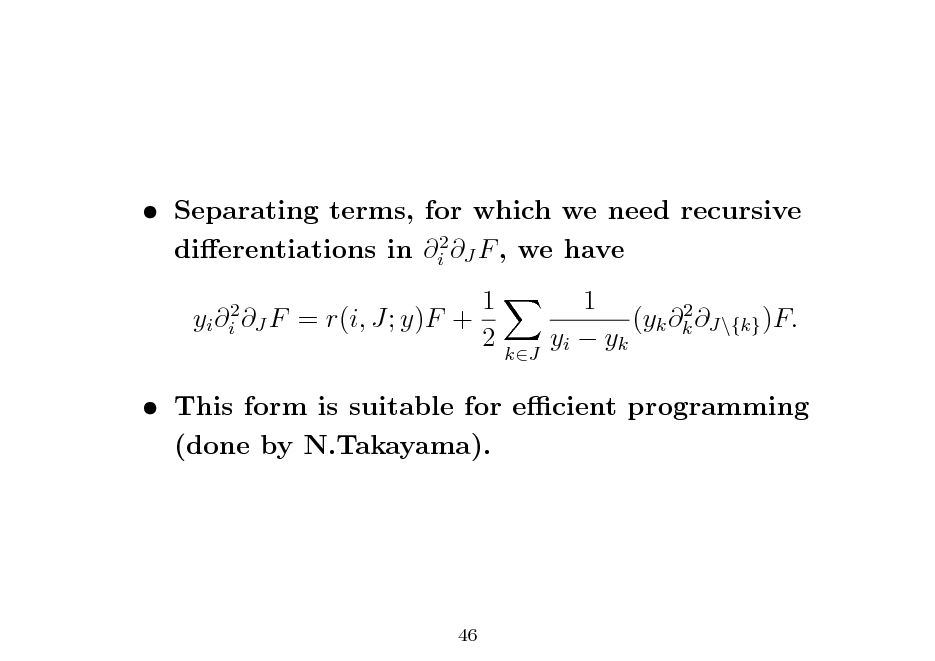Separating terms, for which we need recursive dierentiations in i2 J F , we have yi i2 J F 1 = r(i, J; y)F + 2 1 2 (yk k J\{k} )F. yi yk

kJ

This form is suitable for ecient programming (done by N.Takayama).

46

47When we expand the recursion to the end, we obtain yi i2 J F 1 + 4 1 = r(i, J; y)F + 2k 1 r(k1 , J \ {k}; y)F y i y k1

k1 ,k2 J k1 ,k2 :distinct

1 r(k2 , J \ {k1 , k2 }; y)F (yi yk1 )(yk1 yk2 ) 1 r(k|J| , ; y)F. (yi yk1 ) . . . (yk|J|1 yk|J| )

1 J

+ ... 1 + |J| 2

k1 ,...,k|J| :distinct

k1 ,...,k|J| J

47

48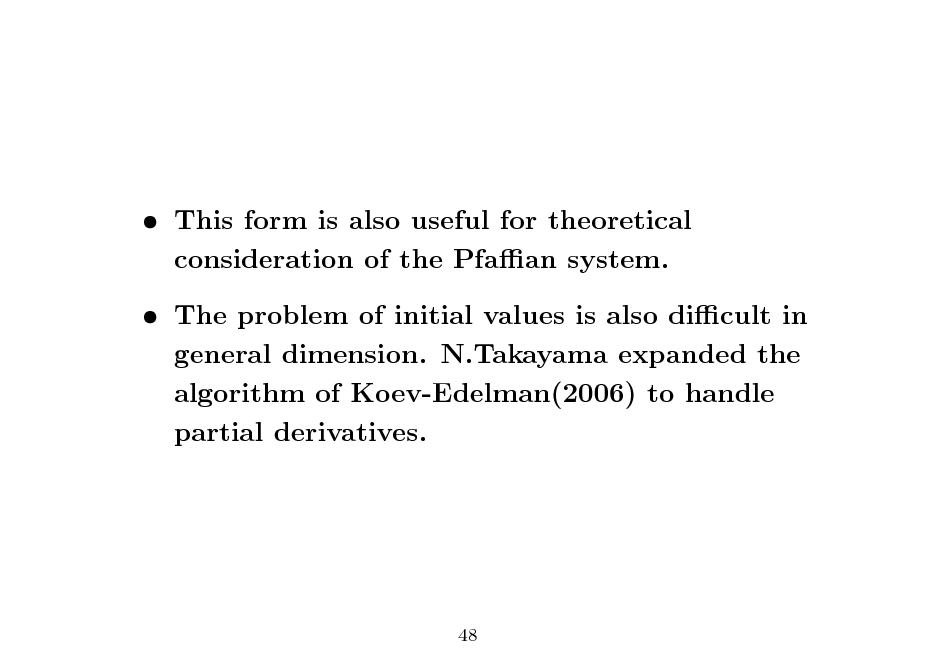This form is also useful for theoretical consideration of the Pfaan system. The problem of initial values is also dicult in general dimension. N.Takayama expanded the algorithm of Koev-Edelman(2006) to handle partial derivatives.

48

49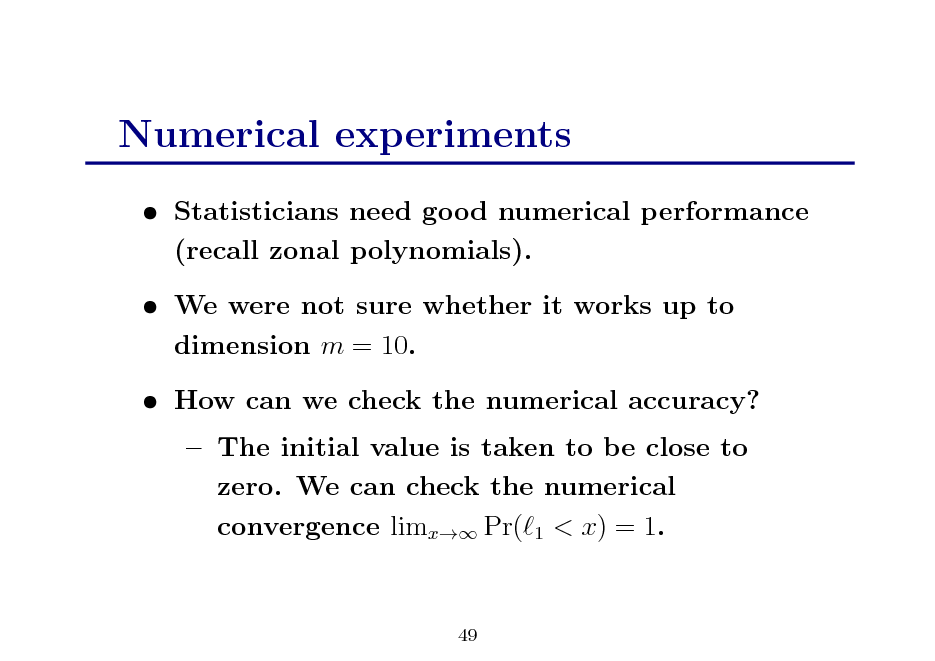Numerical experiments
Statisticians need good numerical performance (recall zonal polynomials). We were not sure whether it works up to dimension m = 10. How can we check the numerical accuracy? The initial value is taken to be close to zero. We can check the numerical convergence limx Pr( 1 < x) = 1.

49

50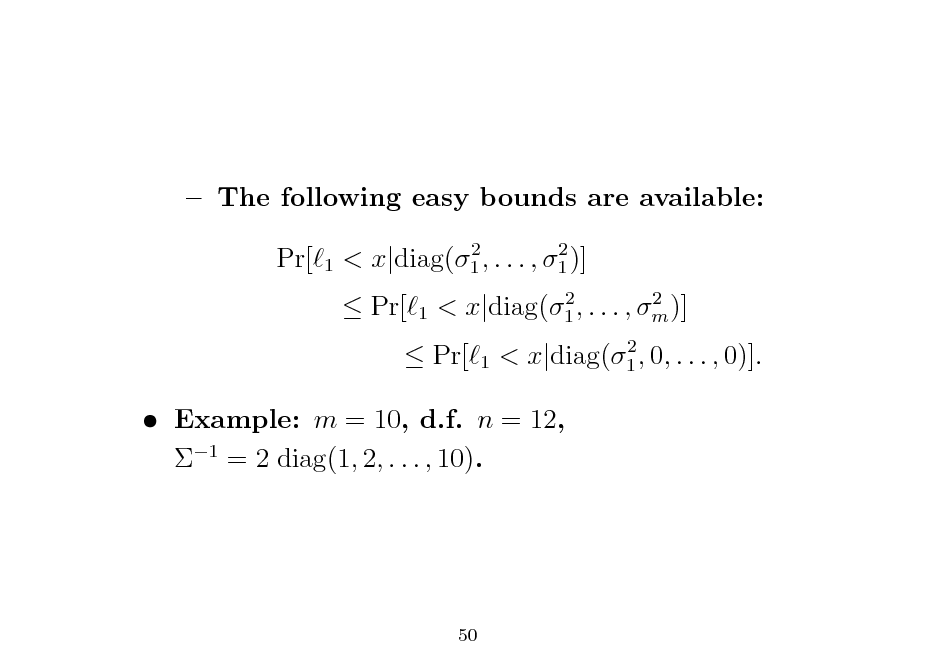The following easy bounds are available: Pr[
1 2 2 < x|diag(1 , . . . , 1 )]

Pr[

1

2 2 < x|diag(1 , . . . , m )] 1 2 < x|diag(1 , 0, . . . , 0)].

Pr[

Example: m = 10, d.f. n = 12, 1 = 2 diag(1, 2, . . . , 10).

50

51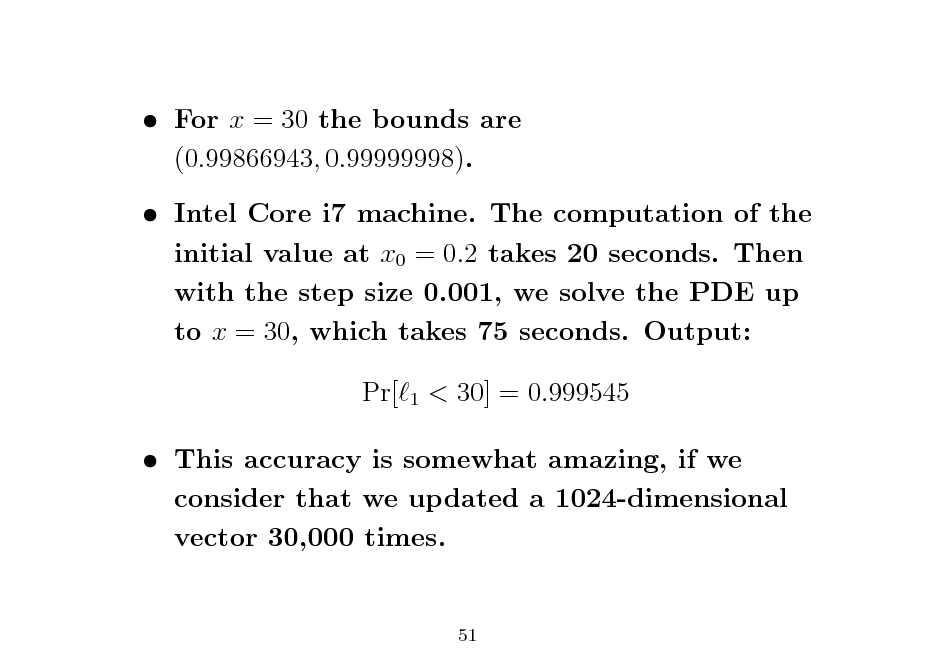For x = 30 the bounds are (0.99866943, 0.99999998). Intel Core i7 machine. The computation of the initial value at x0 = 0.2 takes 20 seconds. Then with the step size 0.001, we solve the PDE up to x = 30, which takes 75 seconds. Output: Pr[
1

< 30] = 0.999545

This accuracy is somewhat amazing, if we consider that we updated a 1024-dimensional vector 30,000 times.

51

52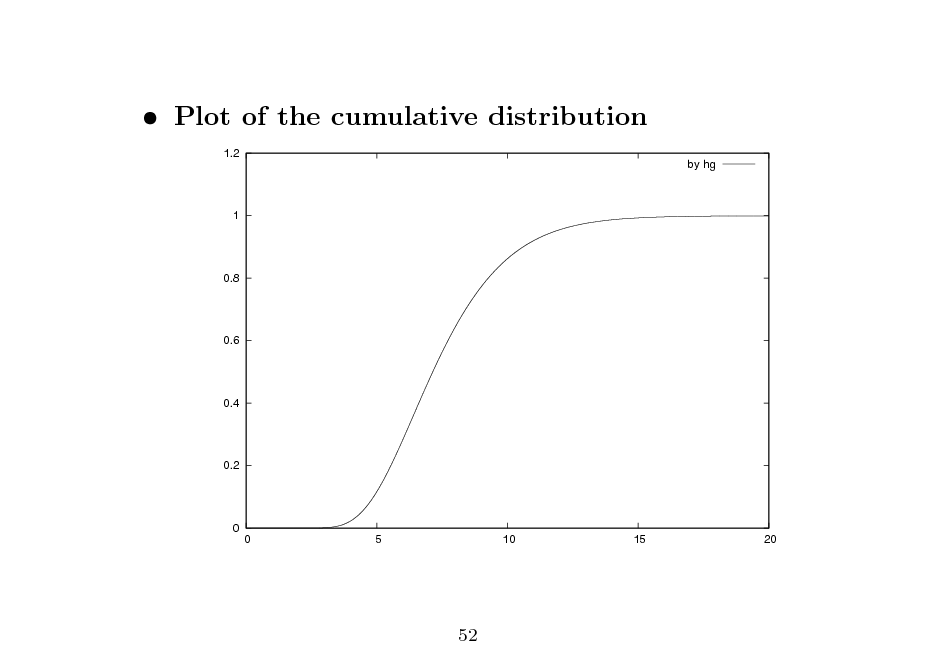Plot of the cumulative distribution
1.2 by hg 1

0.8

0.6

0.4

0.2

0

0

5

10

15

20

52

53Summary
Holonomic gradient method is practical if we implement it eciently. Our approach brought a totally new approach to a longstanding dicult problem in multivariate statistics. Holonomic gradient methods is general and can be applied to many problems. We stand at the beginning of applications of D-module theory to statistics!
53

54